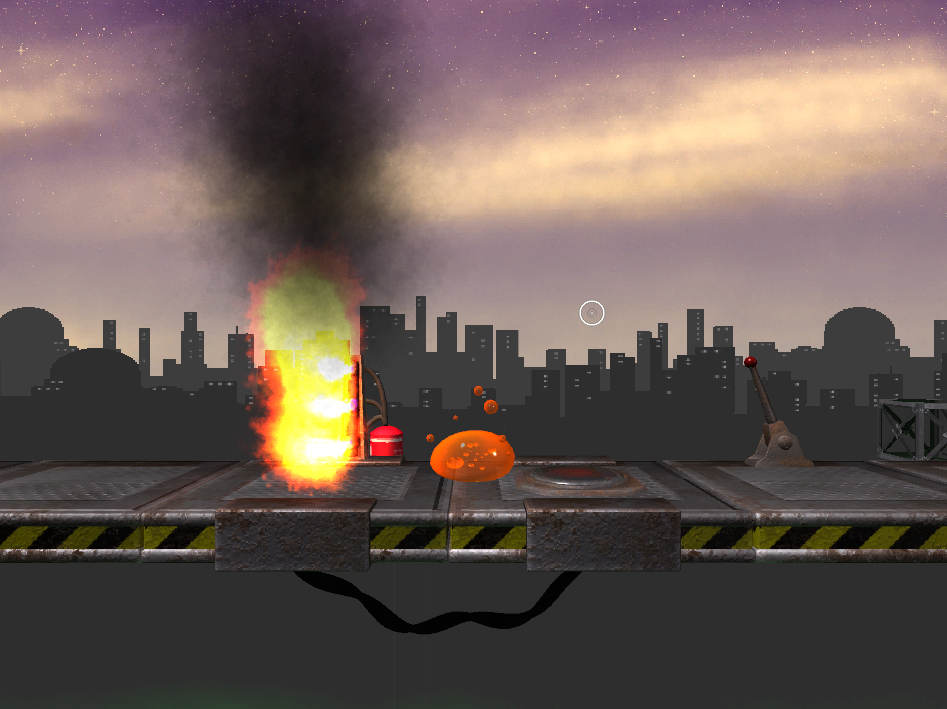## Procedural Splines 2

After trying to wrap my head around procedural mesh generation in Unity for hours and failing repeatedly, I decided to use Unity’s Line Renderer instead of generating a real mesh. Besides being easier by orders of magnitude it also helps keeping the polycount down, while offering good visuals, although it seems to ignore most of my material’s properties. Not sure if I can get this under control. I kept the curves I calculated, and created an empty for each sub-Spline with a Line Renderer Component using the points calculated for the respective subspline.

Here is the finished code. Sadly it doesn’t have the instant feedback in the Editor anymore, but I had to remove the ExecuteInEditMode. The objects created weren’t cleaned up properly when returning from PlayMode. If I find a way to have both, I will update the code.

```
using UnityEngine;
using System.Collections;

public class Spliner : MonoBehaviour {

public GameObject next;
public float gravity;
public int steps;

public int subSplines;
public float frequency;
public float amplitude;

public Material material;

private Vector3 curPosition;
private Vector3 nextPosition;
private Vector3[] mainPointArr = new Vector3;

private Vector3[,] subPointArr = new Vector3[0,0];

private GameObject[] children;

void Start() {

if(next) {
nextPosition = next.transform.position;
curPosition = transform.position;

if(steps < 1) steps= 1;

mainPointArr = new Vector3[steps + 1];

for(int i = 0; i <= steps; i++) {
mainPointArr[i] = DrawCosH(i);
}

if(subSplines < 1) subSplines = 1;

subPointArr = new Vector3[subSplines, steps];

for(int i = 0; i < subSplines; i++) {
DrawSubSpline(i);
}

children = new GameObject[subSplines];

for(int i = 0; i < subSplines; i++) {
children[i] = new GameObject("Child0" + i);
children[i].transform.parent = transform;
temp.SetVertexCount(steps);
temp.material = material;
for(int j = 0; j < steps; j++) {
temp.SetPosition(j, subPointArr[i, j]);
}
}
}
}

public Vector3 DrawCosH(int i) {
Vector3 direction = nextPosition - curPosition;
int locStep = steps - 1;

return curPosition + direction/locStep * i + new Vector3(0, gravity * (CosH((1.0f * i / locStep) - 0.5f) - CosH(0.5f)), 0);
}

float CosH(float t) {
return (Mathf.Exp(t) + Mathf.Exp(-t))/2;
}

void DrawSubSpline(int i) {
float locAmp = amplitude * Random.Range(0.5f, 1.2f) / 10;
float locFreq = frequency * Random.Range(0.8f, 1.2f);
float offset = Random.Range(0.0f, 1.0f);
int cw = (Random.Range(0.0f, 1.0f) > 0.5 ? -1 : 1);

for(int j = 0; j < steps; j++) {
Vector3 direction = mainPointArr[j + 1] - mainPointArr[j];
Quaternion rot = Quaternion.LookRotation(direction);

subPointArr[i, j] = mainPointArr[j] + rot * (Vector3.up * Mathf.Sin(1.0f * j * locFreq / steps + offset) + Vector3.right * cw * Mathf.Cos(1.0f * j * locFreq / steps + offset)) * locAmp;
}
}
}

```

Anyway, here is a picture of how it looks like right now.